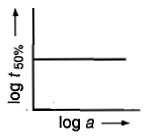# NEET Chemistry Chemical Kinetics Questions Solved

A graph plotted between log (t) 50% vs. log (a) concentration is a straight line. What conclusion can you draw from the given graph?(a) n=1, t1/2 = 1/K.a

(b) n=2, t1/2 = 1/a

(c) n=1, t1/2 = 0.693/K

(d) None of the above